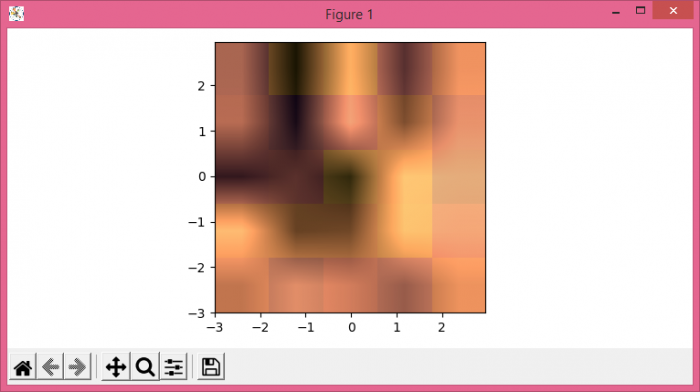# How to plot a layered image in Matplotlib in Python?

To plot a layered image in Matplotlib in Python, we can take the following steps −

• Set the figure size and adjust the padding between and around the subplots.
• Create dx, dy, x, y and extent data using numpy.
• Create a new figure or activate an existing figure using figure() method.
• Create data1 and data2 to display the data as an image, i.e., on a 2D regular raster.
• To display the figure, use show() method.

## Example

import matplotlib.pyplot as plt
import numpy as np

plt.rcParams["figure.figsize"] = [7.50, 3.50]
plt.rcParams["figure.autolayout"] = True

dx, dy = 0.05, 0.05
x = np.arange(-3.0, 3.0, dx)
y = np.arange(-3.0, 3.0, dy)
extent = np.min(x), np.max(x), np.min(y), np.max(y)

fig = plt.figure(frameon=False)

data1 = np.random.rand(5, 5)
plt.imshow(data1, cmap="plasma", interpolation='nearest', extent=extent)

data2 = np.random.rand(5, 5)
plt.imshow(data2, cmap="copper", alpha=.9, interpolation='bilinear', extent=extent)

plt.show()

## Output